### limit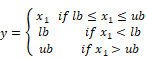Block Inputs: Scalar.

The limit block limits the output signal to a specified upper and lower bound. If the input is less than the lower bound, the limit block limits the output to the lower bound. Similarly, if the input is greater than the upper bound, the limit block limits the output to the upper bound. If the input falls within the specified bounds, the input is transferred to the output unchanged.

The limit block is particularly useful for simulating variables or processes that reach saturation.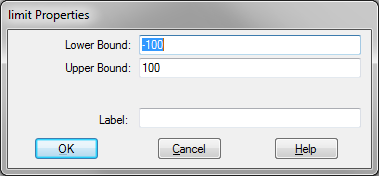Label: Indicates a user-defined block label.

Lower Bound: Indicates the lowest value that the output signal can attain. The default is -100.

Upper Bound: Indicates the highest value that the output signal can attain. The default is 100.

#### Examples

1. Simulation of saturation

Consider a variable y such that: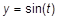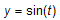Furthermore, assume that y reaches saturation at +0.7 and -0.7. This equation can be realized as shown below.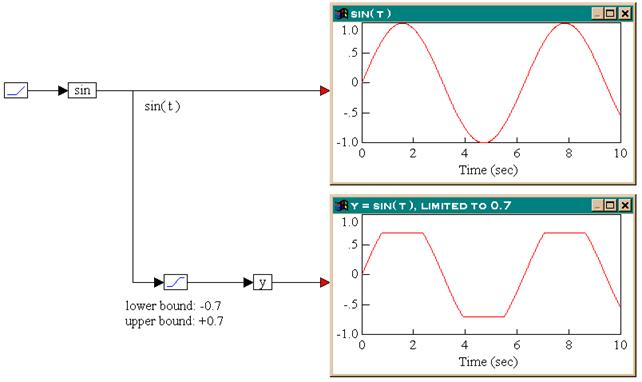From the results in the two plot blocks, the output of the limit block is identical to the input, when the input is within the bounds (-0.7 to +0.7). When the input is out of these bounds, the output is limited to the upper or lower bound values.